$$\newcommand{\xv}{\mathbf{x}} \newcommand{\Xv}{\mathbf{X}} \newcommand{\yv}{\mathbf{y}} \newcommand{\Yv}{\mathbf{Y}} \newcommand{\zv}{\mathbf{z}} \newcommand{\av}{\mathbf{a}} \newcommand{\Wv}{\mathbf{W}} \newcommand{\wv}{\mathbf{w}} \newcommand{\gv}{\mathbf{g}} \newcommand{\Hv}{\mathbf{H}} \newcommand{\dv}{\mathbf{d}} \newcommand{\Vv}{\mathbf{V}} \newcommand{\vv}{\mathbf{v}} \newcommand{\tv}{\mathbf{t}} \newcommand{\Tv}{\mathbf{T}} \newcommand{\Sv}{\mathbf{S}} \newcommand{\zv}{\mathbf{z}} \newcommand{\Zv}{\mathbf{Z}} \newcommand{\Norm}{\mathcal{N}} \newcommand{\muv}{\boldsymbol{\mu}} \newcommand{\sigmav}{\boldsymbol{\sigma}} \newcommand{\phiv}{\boldsymbol{\phi}} \newcommand{\Phiv}{\boldsymbol{\Phi}} \newcommand{\Sigmav}{\boldsymbol{\Sigma}} \newcommand{\Lambdav}{\boldsymbol{\Lambda}} \newcommand{\half}{\frac{1}{2}} \newcommand{\argmax}{\underset{#1}{\operatorname{argmax}}} \newcommand{\argmin}{\underset{#1}{\operatorname{argmin}}} \newcommand{\dimensionbar}{\underset{#1}{\operatorname{|}}}$$

# Introduction to Classification¶

## Classification with Linear Least Squares¶

To classify a sample as being a member of 1 of 3 different classes, we could use integers 1, 2, and 3 as target outputs.Linear function of $x$ seems to match data fairly well. Why is this not a good idea?

We must convert the continuous y-axis value to discrete integers 1, 2, or 3. Without adding more parameters, we are forced to use the general solution of splitting at 1.5 and 2.5.Rats! Boundaries are not where we want them.

## Indicator Variables¶

To allow flexibility, we need to decouple the modeling of the boundaries. Problem is due to using one value to represent all classes. Instead, let's use three values, one for each class. Binary-valued variables are adequate. Class 1 = $(1,0,0)$, Class 2 = $(0,1,0)$ and Class 3 = $(0,0,1)$. These are called indicator variables.

Our linear model has three outputs now. How do we interpret the output for a new sample?

Let the output be $\yv = (y_1, y_2, y_3)$. Convert these values to a class by picking the maximum value.

\begin{align*} \text{class} = \argmax{i}\;\; y_i \end{align*}

We can plot the three output components on three separate graphs. What linear functions will each one learn?Overlay them to see which one is the maximum for each $x$ value.See any potential problems?

What if the green line is too low?What could cause this?

Too few samples from Class 2.There may be no values of $x$ for which the second output, $y_2$, of our linear model is larger than the other two. Class 2 has become masked by the other classes.

What other shape of function response would work better for this data? Hold that thought, while we try an example.

## Example¶

Let's use the parkinsons data set from UCI ML Archive.

• 147 samples from subjects with Parkinsons, 48 samples from healthy subjects
• Each sample composed of 22 numerical features extracted from voice recordings
• Feature named status is 0 for healthy subjects, 1 for subjects with Parkinson's Disease
• from collaboration with the University of Oxford and the National Center for Voice and Speech in Denver.

Let's download the data file and read it in. Also print the shapes of X and T and summarize the X and T data.

In :
import numpy as np
import pandas as pd
import matplotlib.pyplot as plt

In :
import pandas as pd

In :
data.shape

Out:
(195, 24)
In :
data.columns

Out:
Index(['name', 'MDVP:Fo(Hz)', 'MDVP:Fhi(Hz)', 'MDVP:Flo(Hz)', 'MDVP:Jitter(%)',
'MDVP:Jitter(Abs)', 'MDVP:RAP', 'MDVP:PPQ', 'Jitter:DDP',
'MDVP:Shimmer', 'MDVP:Shimmer(dB)', 'Shimmer:APQ3', 'Shimmer:APQ5',
'MDVP:APQ', 'Shimmer:DDA', 'NHR', 'HNR', 'status', 'RPDE', 'DFA',
dtype='object')
In :
T = data['status'].values
T = T.reshape((-1, 1))
Tname = 'status'
T.shape

Out:
(195, 1)
In :
X = data
X = X.drop(['status', 'name'], axis=1)
Xnames = X.columns.tolist()
X = X.values
X.shape, Xnames

Out:
((195, 22),
['MDVP:Fo(Hz)',
'MDVP:Fhi(Hz)',
'MDVP:Flo(Hz)',
'MDVP:Jitter(%)',
'MDVP:Jitter(Abs)',
'MDVP:RAP',
'MDVP:PPQ',
'Jitter:DDP',
'MDVP:Shimmer',
'MDVP:Shimmer(dB)',
'Shimmer:APQ3',
'Shimmer:APQ5',
'MDVP:APQ',
'Shimmer:DDA',
'NHR',
'HNR',
'RPDE',
'DFA',
'D2',
'PPE'])
In :
print(f'{" ":20s} {"mean":9s} {"stdev":9s}')
for i in range(len(Xnames)):
print(f'{Xnames[i]:20s} {np.mean(X[:, i]):9.3g} {np.std(X[:, i]):9.3g}')

                     mean      stdev
MDVP:Fo(Hz)                154      41.3
MDVP:Fhi(Hz)               197      91.3
MDVP:Flo(Hz)               116      43.4
MDVP:Jitter(%)         0.00622   0.00484
MDVP:Jitter(Abs)       4.4e-05  3.47e-05
MDVP:RAP               0.00331   0.00296
MDVP:PPQ               0.00345   0.00275
Jitter:DDP             0.00992   0.00888
MDVP:Shimmer            0.0297    0.0188
MDVP:Shimmer(dB)         0.282     0.194
Shimmer:APQ3            0.0157    0.0101
Shimmer:APQ5            0.0179     0.012
MDVP:APQ                0.0241    0.0169
Shimmer:DDA              0.047    0.0304
NHR                     0.0248    0.0403
HNR                       21.9      4.41
RPDE                     0.499     0.104
DFA                      0.718    0.0552
D2                        2.38     0.382
PPE                      0.207    0.0899

In :
uniq = np.unique(T)
print('   Value  Occurrences')
for i in uniq:
print(f'{i:7.1g} {np.sum(T==i):10d}')

   Value  Occurrences
0         48
1        147


Two indicator variables is equivalent to using single variable, so we will stick with status as our output variable, with value of 0 meaning healthy and 1 meaning Parkinsons.

For small sample size or very uneven number of samples from each class, force equal sampling proportions of two classes when building train, test partitions. Let's use 80% for training and 20% for testing.

In :
trainf = 0.8
healthyI,_ = np.where(T == 0)
parkI,_ = np.where(T == 1)
healthyI = np.random.permutation(healthyI)
parkI = np.random.permutation(parkI)

nHealthy = round(trainf * len(healthyI))
nPark = round(trainf * len(parkI))
rowsTrain = np.hstack((healthyI[:nHealthy], parkI[:nPark]))
Xtrain = X[rowsTrain, :]
Ttrain = T[rowsTrain, :]
rowsTest = np.hstack((healthyI[nHealthy:], parkI[nPark:]))
Xtest =  X[rowsTest, :]
Ttest =  T[rowsTest, :]

print('Xtrain is {:d} by {:d}. Ttrain is {:d} by {:d}'.format(*(Xtrain.shape + Ttrain.shape)))
uniq = np.unique(Ttrain)
print('   Value  Occurrences')
for i in uniq:
print(f'{i:7.1g} {np.sum(Ttrain == i):10d}')

print('Xtest is {:d} by {:d}. Ttest is {:d} by {:d}'.format(*(Xtest.shape + Ttest.shape)))
uniq = np.unique(Ttest)
print('   Value  Occurrences')
for i in uniq:
print(f'{i:7.1g} {np.sum(Ttest == i):10d}')

Xtrain is 156 by 22. Ttrain is 156 by 1
Value  Occurrences
0         38
1        118
Xtest is 39 by 22. Ttest is 39 by 1
Value  Occurrences
0         10
1         29


That's about the same ratio of 0's and 1's.

In :
38/118, 10/29

Out:
(0.3220338983050847, 0.3448275862068966)

and in the original data set we had

In :
48/147

Out:
0.32653061224489793

## Least Squares Solution¶

First let's standardize the inputs. Don't standardize the outputs. They indicate the class. Then just calculate the linear least squares solution.

In :
def train(X, T, lamb=0):
means = X.mean(0)
stds = X.std(0)
n,d = X.shape
Xs = (X - means) / stds
Xs1 = np.insert(Xs , 0, 1, axis=1)
lambDiag = np.eye(d + 1) * lamb
lambDiag[0, 0] = 0
w = np.linalg.lstsq( Xs1.T @ Xs1 + lambDiag, Xs1.T @ T, rcond=None)
return {'w': w, 'means':means, 'stds':stds}

def use(model, X):
Xs = (X - model['means']) / model['stds']
Xs1 = np.insert(Xs , 0, 1, axis=1)
return Xs1 @ model['w']

In :
Xnames

Out:
['MDVP:Fo(Hz)',
'MDVP:Fhi(Hz)',
'MDVP:Flo(Hz)',
'MDVP:Jitter(%)',
'MDVP:Jitter(Abs)',
'MDVP:RAP',
'MDVP:PPQ',
'Jitter:DDP',
'MDVP:Shimmer',
'MDVP:Shimmer(dB)',
'Shimmer:APQ3',
'Shimmer:APQ5',
'MDVP:APQ',
'Shimmer:DDA',
'NHR',
'HNR',
'RPDE',
'DFA',
'D2',
'PPE']
In :
model = train(Xtrain, Ttrain)

Xnames.insert(0,'bias')
for i in range(len(Xnames)):
print('{:2d} {:>20s} {:10.3g}'.format(i, Xnames[i], model['w'][i]))

 0                 bias      0.756
1          MDVP:Fo(Hz)     -0.123
2         MDVP:Fhi(Hz)    -0.0165
3         MDVP:Flo(Hz)    -0.0513
4       MDVP:Jitter(%)     -0.975
5     MDVP:Jitter(Abs)     -0.141
6             MDVP:RAP       -2.1
7             MDVP:PPQ    -0.0746
8           Jitter:DDP       3.18
9         MDVP:Shimmer       0.66
10     MDVP:Shimmer(dB)     0.0779
11         Shimmer:APQ3        -56
12         Shimmer:APQ5     -0.313
13             MDVP:APQ    -0.0912
14          Shimmer:DDA       55.7
15                  NHR      -0.11
16                  HNR    -0.0908
17                 RPDE     -0.146
18                  DFA   -0.00457
21                   D2   -0.00708
22                  PPE      0.107


Which ones appear to be most important?

And, of course, let's test our linear model. To compare to the target values of 0 and 1, we must convert the continuous output value to 0 or 1, whichever is closest.

In :
def convertTo01(Y):
distFromTarget = np.abs(Y - [0,1])
whichTargetClosest = np.argmin(distFromTarget, axis=1).reshape((-1, 1))
return whichTargetClosest  # column index equivalent to 0 and 1 targets

In :
convertTo01(np.array([0.1, 1.1, -0.5, 0.56]).reshape((-1,1)))

Out:
array([,
,
,
])
In :
Ytrain = use(model, Xtrain)

predictedTrain = convertTo01(Ytrain)

percentCorrectTrain = np.sum(predictedTrain == Ttrain) / Ttrain.shape * 100.0

Ytest = use(model, Xtest)

predictedTest = convertTo01(Ytest)
percentCorrectTest = np.sum(predictedTest == Ttest) / float(Ttest.shape) * 100.0

print('Percent Correct: Training {:6.1f} Testing {:6.1f}'.format(percentCorrectTrain, percentCorrectTest))

Percent Correct: Training   91.0 Testing   94.9


What visualization would you use to check the results?

Let's plot the true class with the output of the model for each training sample, then each testing

In :
plt.figure(figsize=(8, 8))
plt.subplot(2, 1 ,1)
plt.plot(np.hstack((Ttrain, predictedTrain)), 'o-', alpha=0.5)
plt.ylim(-0.1, 1.1) # so markers will show
plt.xlabel('Sample Index')
plt.ylabel('Class')
plt.title('Training Data')
plt.legend(('Actual', 'Predicted'), loc='center')

plt.subplot(2, 1, 2)
plt.plot(np.hstack((Ttest, predictedTest)), 'o-', alpha=0.5)
plt.ylim(-0.1, 1.1)
plt.xlabel('Sample Index')
plt.ylabel('Class')
plt.title('Testing Data')
plt.legend(('Actual', 'Predicted'), loc='center');

plt.tight_layout()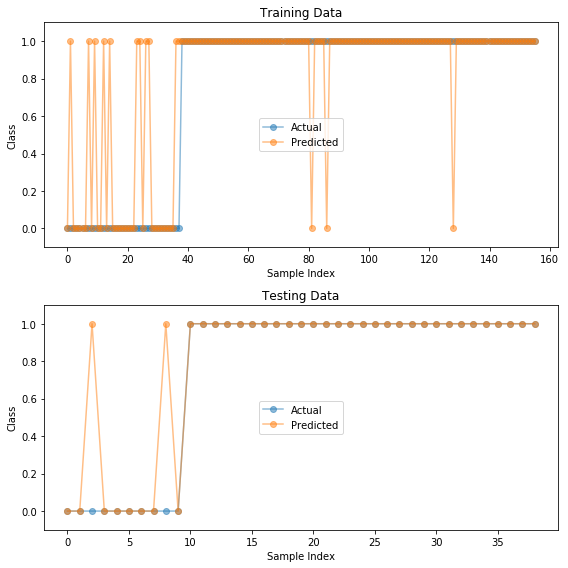Might also be revealing to add the continuously-valued output of the network, before being converted to the class.

In :
plt.figure(figsize=(8, 8))

plt.subplot(2, 1, 1)
plt.plot(np.hstack((Ttrain, predictedTrain, Ytrain)),'o-', alpha=0.5)
plt.ylim(-0.1, 1.1) # so markers will show
plt.xlabel('Sample Index')
plt.ylabel('Class')
plt.title('Training Data')
plt.legend(('Actual', 'Predicted', 'Cont. Val.'), loc='center')

plt.subplot(2, 1, 2)
plt.plot(np.hstack((Ttest, predictedTest, Ytest)), 'o-', alpha=0.5)
plt.ylim(-0.1, 1.1)
plt.xlabel('Sample Index')
plt.ylabel('Class')
plt.title('Testing Data')
plt.legend(('Actual', 'Predicted', 'Cont. Val.'), loc='center');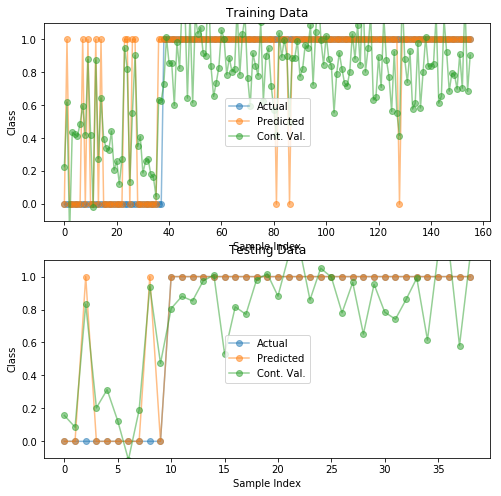## Shape of Boundary¶

Imagine we have just two variable attributes, $x_1$ and $x_2$. With our linear least squared model $\wv$, we make a prediction for sample $\xv=(x_1,x_2)$ by

$$y(\xv) = w_0 + w_1 x_1 + w_2 x_2$$

For the parkinsons problem, we will predict the class for this sample is 'healthy' if

$$y(\xv) = w_0 + w_1 x_1 + w_2 x_2 < 0.5$$

So the boundary between the 'healthy' and the 'parkinsons' class in the two-dimensional $x_1, x_2$ space

$$w_0 + w_1 x_1 + w_2 x_2 = 0.5$$

is of what shape?

Above methods are discriminative in nature, meaning that what is learned is a function that is designed to produce different values for different classes.

An alternative approach is to first create a probabilistic model of samples from each class, forming a model with which samples from a class can be generated, hence a generative model. The number of models is the same as the number of classes.

Before jumping into the details of simple generative models, we will first review probability theory, joint probabilities, conditional probabilities, Bayes theorem, and the Gaussian distribution.

# Probability Theory¶

## Boxes of Fruit¶Counts of fruit in each jar:

. apples oranges strawberries Sums
red jar 2 6 4 $\Sigma$ = 12
blue jar 3 1 2 $\Sigma$ = 6

Probabilities of fruit from a given jar:

. apples oranges strawberries Sums
red jar 2/12 = 0.167 6/12 = 0.5 4/12 = 0.33 $\Sigma$ = 1.0
blue jar 3/6 = 0.5 1/6 = 0.167 2/6 = 0.33 $\Sigma$ = 1.0

Say the probability of choosing the red jar is 0.6 and for choosing the blue jar is 0.4. The probability of choosing the red jar and drawing an apple out of the red jar is the product of these two choices, or $0.6 (0.167) = 0.1$.

Doing all multiplications results in

. apples oranges strawberries Sums
red jar (P=0.6) 0.6(0.167) = 0.1 0.6(0.5) = 0.3 0.6(0.33) = 0.2 $\Sigma$ = 0.5982
blue jar (P=0.4) 0.4(0.5) = 0.2 0.4(0.167) = 0.067 0.4(0.33) = 0.133 $\Sigma$ = 0.3988

## Joint Probability Table¶

Combine in a two-dimensional table to show joint probabilities of two events.

. . . fruit . .
. . apple orange strawberry Sums
jar red 0.1 0.3 0.2 $\Sigma$ = 0.5982
. blue 0.2 0.067 0.133 $\Sigma$ = 0.3988
. Sums $\Sigma$=0.3 $\Sigma$ = 0.367 $\Sigma$ = 0.333 $\Sigma$=1

Symbolically, let $J$ be a random variable for jar, and $F$ be a random variable for fruit:

. . . fruit . .
. . apple orange strawberry Sums
jar red P(J=red,F=apple) P(J=red,F=orange) P(J=red,F=strawberry) P(J=red)
. blue P(J=blue,F=apple) P(J=blue,F=orange) P(J=blue,F=strawberry) P(J=blue)
. Sums P(F=apple) P(F=orange) P(F=strawberry) 1

## Bayes Rule¶

Just saw an example of the product rule:

\begin{align*} P(F=orange, J=blue) = P(F=orange | J=blue) P(J=blue) \end{align*}

Since

\begin{align*} P(F=orange, J=blue) = P(J=blue, F=orange) \end{align*}

and

\begin{align*} P(J=blue,F=orange) = P(J=blue|F=orange)P(F=orange) \end{align*}

we know

\begin{align*} P(J=blue|F=orange)& P(F=orange) =\\ & P(F=orange | J=blue) P(J=blue) \end{align*}

Dividing both sides by $P(F=orange)$ leads to Bayes Rule:

\begin{align*} P(J=blue | F=orange) = \frac{P(F=orange|J=blue)P(J=blue)}{P(F=orange)} \end{align*}

On the right hand side of Bayes Rule, all terms are given except $P(F=orange)$

\begin{align*} P(J=blue | F=orange) = \frac{P(F=orange|J=blue)P(J=blue)}{P(F=orange)} \end{align*}

We can use the sum rule to get this

\begin{align*} P(F=orange) & = \sum_{j\in\{red,blue\}} P(F=orange,J=j) \\ & = 0.3+0.067\\ & = 0.367 \end{align*}

So, Bayes Rule can be rewritten as

\begin{align*} P(J=blue|F=orange) = \frac{P(F=orange|J=blue)P(J=blue)}{\sum_j P(F=orange,J=j)} \end{align*}

or

\begin{align*} P(J=blue|F=orange) = \frac{P(F=orange|J=blue)P(J=blue)}{\sum_j P(F=orange|J=j)P(J=j)} \end{align*}

Now in Python. We can represent a conditional probability table as a two-dimensional array.

In :
counts = np.array([[2, 6, 4], [3, 1, 2]])
counts

Out:
array([[2, 6, 4],
[3, 1, 2]])

Let's include the row and column names as lists, and write a function to print the table.

In :
jarNames = ['red', 'blue']
fruitNames = ['apple', 'orange', 'strawberry']

In :
def printTable(label, data):
print
print(label)
print('   {:>9s} {:>7s} {:>9s}'.format(*fruitNames))
for i in [0, 1]:
d = data[i, :].tolist()
print('{:4s} {:7.3g} {:7.3g} {:7.3g} {:7.3g}'.format(*([jarNames[i]] + d + [sum(d)])))
colTotals = np.sum(data, axis=0).tolist()
print('     {:7.3g} {:7.3g} {:7.3g} {:7.3g}'.format(*(colTotals + [sum(colTotals)])))

printTable('counts', counts)

counts
apple  orange strawberry
red        2       6       4      12
blue       3       1       2       6
5       7       6      18


Calculate the sums of fruits in each jar by

In :
jarSums = np.sum(counts, axis=1).reshape((2, 1))
jarSums

Out:
array([,
[ 6]])

Now we can calculate the probability of drawing each type of fruit, given that we have already chosen a jar.

In :
pFruitGivenJar = counts / jarSums
printTable('Prob(Fruit|Jar)', pFruitGivenJar)

Prob(Fruit|Jar)
apple  orange strawberry
red    0.167     0.5   0.333       1
blue     0.5   0.167   0.333       1
0.667   0.667   0.667       2


We can do more if we code the probability of selecting a jar.

In :
pJar = np.array([[0.6], [0.4]])
pJar

Out:
array([[0.6],
[0.4]])

Now we can calculate the joint probabilities, or the probabilities of each pair of a jar and a fruit occurring.

In :
pFruitAndJar = pFruitGivenJar * pJar
printTable('Prob(Fruit,Jar)', pFruitAndJar)

Prob(Fruit,Jar)
apple  orange strawberry
red      0.1     0.3     0.2     0.6
blue     0.2  0.0667   0.133     0.4
0.3   0.367   0.333       1


The sum at the lower right had better be 1, because this table is all possible results.

How do we get the probability of a fruit from this table? Just sum over the jars to marginalize out (remove) the jars.

In :
pFruit = np.sum(pFruitAndJar, axis=0)
pFruit

Out:
array([0.3       , 0.36666667, 0.33333333])

Now the probability of a jar given that you know which fruit was drawn, is

In :
pJarGivenFruit = pFruitAndJar / pFruit
printTable('Prob(Jar|Fruit)', pJarGivenFruit)

Prob(Jar|Fruit)
apple  orange strawberry
red    0.333   0.818     0.6    1.75
blue   0.667   0.182     0.4    1.25
1       1       1       3


# Bayes Rule for Classification¶

Replace jars with groups of hand-drawn digits. Replace fruits with hand-drawn images.Let $i$ be a particular image. To classify an image $i$ as a particular digit, such as 4, we want to know

\begin{align*} P(Digit = 4 \;|\; Image = i) \end{align*}

but we probably only know

\begin{align*} P(Image = i \;|\; Digit = 4) \end{align*}

If we assume

\begin{align*} P(Image=i) = &\frac{1}{\mbox{number of images}}\\ P(Digit=4)=&\frac{1}{10} \end{align*}

then we can use Bayes rule:

\begin{align*} P(Digit=4\;|\;Image=i) & = \frac{P(Image=i\;|\;Digit=4) P(Digit=4)}{P(Image=i)}\\ &\\ & = \frac{P(Image=i\;|\;Digit=4) 0.1}{(1/\mbox{number of images)}} \end{align*}

# Classification: Simple Generative Models¶

Above we discussed a linear function as a discriminant function. If we had three classes, we would have three discriminant functions, and their values would be compared to find the maximum value to make the class prediction.

A different way to develop a similar comparison is to start with models of the data from each class. If the models define a probability distribution over possible values, the models are generative models.

What shape model would you like?

## First, Why Gaussians?¶

$\newcommand{\xv}{\mathbf{x}} \newcommand{\Xv}{\mathbf{X}} \newcommand{\piv}{\mathbf{\pi}} \newcommand{\yv}{\mathbf{y}} \newcommand{\Yv}{\mathbf{Y}} \newcommand{\zv}{\mathbf{z}} \newcommand{\av}{\mathbf{a}} \newcommand{\Wv}{\mathbf{W}} \newcommand{\wv}{\mathbf{w}} \newcommand{\gv}{\mathbf{g}} \newcommand{\Hv}{\mathbf{H}} \newcommand{\dv}{\mathbf{d}} \newcommand{\Vv}{\mathbf{V}} \newcommand{\vv}{\mathbf{v}} \newcommand{\tv}{\mathbf{t}} \newcommand{\Tv}{\mathbf{T}} \newcommand{\Sv}{\mathbf{S}} \newcommand{\zv}{\mathbf{z}} \newcommand{\Zv}{\mathbf{Z}} \newcommand{\Norm}{\mathcal{N}} \newcommand{\muv}{\boldsymbol{\mu}} \newcommand{\sigmav}{\boldsymbol{\sigma}} \newcommand{\phiv}{\boldsymbol{\phi}} \newcommand{\Phiv}{\boldsymbol{\Phi}} \newcommand{\Sigmav}{\boldsymbol{\Sigma}} \newcommand{\Lambdav}{\boldsymbol{\Lambda}} \newcommand{\half}{\frac{1}{2}} \newcommand{\argmax}{\underset{#1}{\operatorname{argmax}}} \newcommand{\argmin}{\underset{#1}{\operatorname{argmin}}} \newcommand{\dimensionbar}{\underset{#1}{\operatorname{|}}}$

How would you like to model the probability distribution of a typical cluster of your data? If, and that's a big if, you believe the data samples from a particular class have attribute values that tend to be close to a particular value, that is, that the samples cluster about a central point in the sample space, then pick a probabilistic model that has a peak over that central point and falls towards zero as you move away from that point.

How do we construct such a model? Well, let's try for two characteristics:

• The model's value will decrease with the distance from the central point, and
• its value will always be greater than 0. If $\xv$ is a sample and $\muv$ is the central point, we can achieve this with $$p(\xv) = \frac{1}{||\xv - \muv||}$$ where $||\xv - \muv||$ is the distance between $\xv$ and $\muv$.

Let's try making a plot of this for $\mu = 5.5$.

In :
import numpy as np
import matplotlib.pyplot as plt

In :
xs = np.linspace(-5,10,1000)
mu = 5.5
plt.plot(xs, 1/np.sqrt((xs-mu)**2))
plt.ylim(0,20)
plt.plot([mu, mu], [0, 20], 'r--',lw=2)
plt.xlabel('$x$')
plt.ylabel('$p(x)$');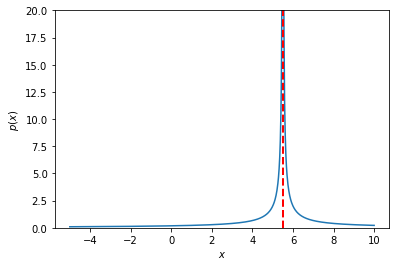The red dotted line is at $\mu = 5.5$.

Humm...meets our criteria, but has problems---goes to infinity at the center and we cannot control the width of the central area where samples may appear.

Can take care of first issue by using the distance as an exponent, so that when it is zero, the result is 1. Let's try a base of 2. $$p(\xv) = \frac{1}{2^{||\xv - \muv||}}$$

Now, let's see...how do we do a calculation with a scalar base and vector exponent? For example, we want $$2^{[2,3,4]} = [2^2, 2^3, 2^4]$$

In :
2**[2,3,4]

---------------------------------------------------------------------------
TypeError                                 Traceback (most recent call last)
<ipython-input-3-f7409be697fa> in <module>
----> 1 2**[2,3,4]

TypeError: unsupported operand type(s) for ** or pow(): 'int' and 'list'

Nope. Maybe we have to use a numpy array.

In :
2**np.array([2,3,4])

Out:
array([ 4,  8, 16])

Hey! That's it.

In :
plt.plot(xs, 1/2**np.sqrt((xs-mu)**2))
plt.plot([mu, mu], [0, 1], 'r--',lw=3)
plt.xlabel('$x$')
plt.ylabel('$p(x)$');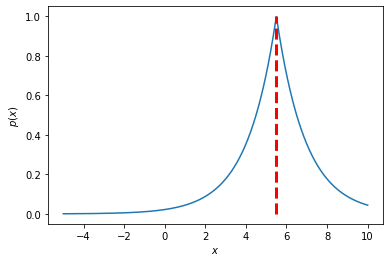Solves the infinity problem, but it still falls off too fast. Want to change the distance to a function that changes more slowly at first, when you are close to the center. How about the square function?
$$p(\xv) = \frac{1}{2^{||\xv - \muv||^2}}$$

In :
plt.plot(xs, 1/2**(xs-mu)**2)
plt.plot([mu, mu], [0, 1], 'r--',lw=3)
plt.xlabel('$x$')
plt.ylabel('$p(x)$');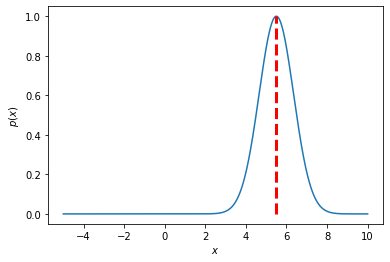Yeah. That's a nice shape. Now we can vary the width by scaling the squared distance. $$p(\xv) = \frac{1}{2^{0.1\,||\xv - \muv||^2}}$$

In :
plt.plot(xs, 1/2**(0.1 * (xs-mu)**2))
plt.plot([mu, mu], [0, 1], 'r--',lw=3)
plt.xlabel('$x$')
plt.ylabel('$p(x)$');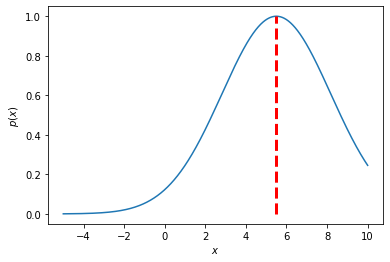There. That's good enough. We could be happy with this. Just pick the center and scale factor that best matches the sample distributions. But, let's make one more change that won't affect the shape of our model, but will simplify later calculations. We will soon see that logarithms come into play when we try to fit our model to a bunch of samples. What is the logarithm of $2^{0.1\,|\xv - \muv|^2}$, or, more simply, the logarithm of $2^z$? If we are talking base 10 logs, $\log 2^z = z \log 2$. Since we are free to pick the base...hey, how about using $e$ and using natural logarithms? Then $\ln e^z = z \ln e = z$. So much simpler! :-)

So, our model is now $$p(\xv) = \frac{1}{e^{0.1\,||\xv - \muv||^2}}$$ which can also be written as $$p(\xv) = e^{-0.1\,||\xv - \muv||^2}$$

In :
plt.plot(xs, np.exp(-0.1 * (xs-mu)**2))
plt.plot([mu, mu], [0, 1], 'r--',lw=3)
plt.xlabel('$x$')
plt.ylabel('$p(x)$');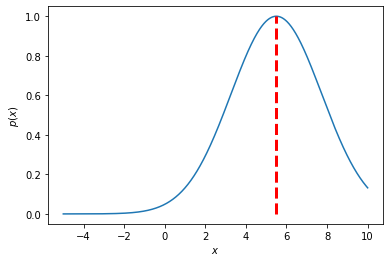The scale factor 0.1 is a bit counterintuitive. The smaller the value, the more spread out our model is. So, let's divide by the scale factor rather than multiply by it, and let's call it $\sigma$. Let's also put it inside the square function, so $\sigma$ is directly scaling the distance, rather than the squared distance.
$$p(\xv) = e^{-\left (\frac{||\xv - \muv||}{\sigma}\right )^2}$$ or $$p(\xv) = e^{-\frac{||\xv - \muv||^2}{\sigma^2}}$$

Speaking of dividing, and this won't surprise you, since we will be taking derivatives of this function with respect to parameters like $\mu$, let's multiply by $\frac{1}{2}$ so that when we bring the exponent 2 down it will cancel with $\frac{1}{2}$. $$p(\xv) = e^{-\frac{1}{2}\frac{||\xv - \muv||^2}{\sigma^2}}$$

One remaining problem we have with our "probabilistic" model is that it is not a true probability distribution, which must

• have values between 0 and 1, $0 \le p(x) \le 1$, and
• have values that sum to 1 over the range of possible $x$ values, $\int_{-\infty}^{+\infty} p(x) dx = 1$.

We have satisfied the first requirement, but not the second. We can fix this by calculating the value of the integral and dividing by that value, which is called the normalizing constant. The value of the integral turns out to be $\sqrt{2\pi\sigma^2}$. See Exercise 1.7, and its solution available at the textbook's website.

So, finally, we have the definition $$p(\xv) = \frac{1}{\sqrt{2\pi\sigma^2}} e^{-\frac{1}{2}\frac{||\xv - \muv||^2}{\sigma^2}}$$ and, TA DA..., we have arrived at the Normal, or Gaussian, probability distribution (technically the density function) with mean $\muv$ and standard deviation $\sigma$, and thus variance $\sigma^2$. Check out the Wikipedia entry.

Now you know a bit about why the Normal distribution is so prevalent. For additional insight and history, read Chapter 7: The Central Gaussian, or Normal, Distribution of Probability Theory: The Logic of Science by E.T. Jaynes, 1993. It starts with this quotation from Augustus de Morgan (yes, that de Morgan) from 1838:

"My own impression...is that the mathematical results have outrun their interpretation and that some simple explanation of the force and meaning of the celebrated integral...will one day be found...which will at once render useless all the works hitherto written."

Before wrestling with python, we need to define the multivariate Normal distribution. Let's go to two dimensions, to make sure we develop code to handle multidimensional data, not just scalars. Now our hill we have been drawing will be a mound up above a two-dimensional base plane. We will define $\xv$ and $\muv$ to be two-dimensional column vectors. What will $\sigma$ be? Well, we need scale factors for the two dimensions to stretch or shrink the mound in the directions of the two base-plane axes. We also need another scale factor to allow the mound to be stretched in directions not parallel to an axis.

Remember, the Normal distribution is all about squared distance from the mean. In two dimensions, the difference vector is $\dv = \xv - \muv = (d_1,d_2)$. The squared distance is therefore $||\dv||^2 = d_1^2 + 2 d_1 d_2 + d_2^2$. Now we see where the three scale factors go: $s_1 d_1^2 + 2 s_2 d_1 d_2 + s_3 d_2^2$. This can be written in matrix form if we collect the scale factors in the matrix $$\Sigmav = \begin{bmatrix} s_1 & s_2\\ s_2 & s_3 \end{bmatrix}$$ so that $$s_1 d_1^2 + 2 s_2 d_1 d_2 + s_3 d_2^2 = \dv^T \Sigmav \dv$$ because \begin{align*} \dv^T \Sigmav \dv & = \begin{bmatrix} d_1 & d_2 \end{bmatrix} \begin{bmatrix} s_1 & s_2\\ s_2 & s_3 \end{bmatrix} \begin{bmatrix} d_1\\ d_2 \end{bmatrix}\ & = \begin{bmatrix} d_1 s_1 + d_2 s_2 & d_1 s_2 + d_2 s_3 \end{bmatrix} \begin{bmatrix} d_1\\ d_2 \end{bmatrix}\ &= # (d_1 s_1 + d_2 s_2) d_1 + (d_1 s_2 + d_2 s_3) d_2 ¶ s_1 d_1^2 + 2 s_2 d_1 d_2 + s_3 d_2^2 \end{align*}

Again, it is more intuitive to use scale factors that divide the distance components rather than multiply them. In the multidimensional world, this means that instead of multiplying by $\Sigmav$ we will multiply by $\Sigmav^{-1}$.

The normalizing constant is a bit more complicated. It involves the determinant of $\Sigmav$, which is the sum of its eigenvalues and can be thought of as a generalized scale factor. Skim through the Wikipedia entry on determinants. The multivariate $D$-dimensional Normal distribution is $$p(\xv) = \frac{1}{(2\pi)^{d/2} |\Sigmav |^{1/2}} e^{-\frac{1}{2} (\xv-\muv)^T \Sigmav^{-1} (\xv - \muv)}$$ where mean $\muv$ is a $D$-dimensional column vector and covariance matrix $\Sigmav$ is a $D\times D$ symmetric matrix.

So, a Gaussian, or Normal distribution, is a nice choice. Its integral sums to one, its value is always nonnegative, and the derivative of its natural logarithm is very nice.

$$p(\xv) = \frac{1}{(2\pi)^{d/2} |\Sigmav |^{1/2}} e^{-\frac{1}{2} (\xv-\muv)^T \Sigmav^{-1} (\xv - \muv)}$$

where mean $\muv$ is a $d$-dimensional column vector and covariance matrix $\Sigmav$ is a $d\times d$ symmetric matrix.

The Normal distribution is also called the Gaussian distribution. (When did Gauss live?)

In addition to the above reasons for concocting this distribution, it has a number of interesting analytical properties. One is the Central Limit Theorem, which states that the sum of many choices of $N$ random variables tends to a Normal distribution as $N \rightarrow \infty$.

Let's play with this theorem with some fancy shmansy python using the new ipython notebook interact feature to explore the distribution of sums as the number of samples varies.

In :
from ipywidgets import interact
maxSamples = 100
nSets = 10000
values = np.random.uniform(0,1,(maxSamples,nSets))

@interact(nSamples=(1,maxSamples))
def sumOfN(nSamples=1):
sums = np.sum(values[:nSamples,:],axis=0)
plt.hist(sums, 20, facecolor='green')


Now how would you check our definition of $p(x)$ in python? First, we need a function to calculate $p(x)$ given $\mu$ and $\Sigma$, or $p(x|\mu, \Sigma)$.

In :
def normald(X, mu, sigma):
""" normald:
X contains samples, one per row, N x D.
mu is mean vector, D x 1.
sigma is covariance matrix, D x D.  """
D = X.shape
detSigma = sigma if D == 1 else np.linalg.det(sigma)
if detSigma == 0:
raise np.linalg.LinAlgError('normald(): Singular covariance matrix')
sigmaI = 1.0/sigma if D == 1 else np.linalg.inv(sigma)
normConstant = 1.0 / np.sqrt((2*np.pi)**D * detSigma)
diffv = X - mu.T # change column vector mu to be row vector
return normConstant * np.exp(-0.5 * np.sum(np.dot(diffv, sigmaI) * diffv, axis=1))[:,np.newaxis]

In :
normald?


Let's check the shapes of matrices in that last calculation.

diffv = X   -  mu.T
|  NxD    Dx1 |
|             |
|            1xD
|
NxD

normConstant * np.exp(-0.5 * np.sum(np.dot(diffv, sigmaI) * diffv, axis=1))[:,newaxis]
1x1                                      NxD    DxD  |    NxD |       |           |
|        |       |           |
NxD      NxD      |           |
|           |
N           |
Nx1



So we get $N$ answers, one for each sample.

In :
np.array([[1,2,3]]).shape

Out:
(1, 3)
In :
X = np.array([[1,2],[3,5],[2.1,1.9]])
mu = np.array([,])
Sigma = np.array([[1,0],[0,1]])
print(X)
print(mu)
print(Sigma)
normald(X, mu, Sigma)

[[1.  2. ]
[3.  5. ]
[2.1 1.9]]
[
]
[[1 0]
[0 1]]

Out:
array([[0.09653235],
[0.00107238],
[0.15757132]])

Okay, but to really see if it is working, let's do some plotting! For two-dimensional samples, we need to make a surface plot in three dimensions to show the value of normald. Find examples of 3D plots in this set of example notebooks.

In :
x = np.linspace(-5, 5, 50)
y = x.copy()
xmesh, ymesh = np.meshgrid(x, y)
xmesh.shape, ymesh.shape

Out:
((50, 50), (50, 50))
In :
X = np.vstack((xmesh.flat, ymesh.flat)).T
X.shape

Out:
(2500, 2)
In :
from mpl_toolkits.mplot3d import Axes3D
from matplotlib import cm

fig = plt.figure(figsize=(15, 10))
ax = fig.gca(projection='3d')
# ax.set_aspect("equal")

mu = np.array([[2,-2]]).T
Sigma = np.array([[1,0],[0,1]])

Z = normald(X, mu, Sigma)
Zmesh = Z.reshape(xmesh.shape)
surface = ax.plot_surface(xmesh, ymesh, Zmesh, rstride=1, cstride=1, cmap=cm.coolwarm, linewidth=0, antialiased=False);

plt.colorbar(surface, shrink=0.3);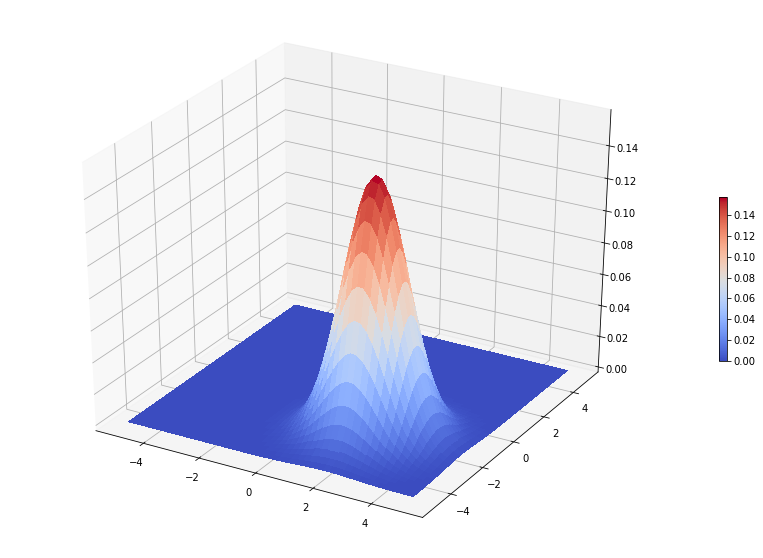Back to that Masking Problem.. What function shape were you thinking of that might fix the masking problem?

Radial basis function? Good choice! But, remember what a radial basis function resembles?

Right again! A Normal distribution.

So, let's say we come up with the generative distribution, such as a Normal distribution, for Class $k$, called $p(\xv|Class=k)$, or $p(\xv|C=k)$. How do we use it to classify?

Can just take the distribution with the highest value, $\argmax{k}\; p(\xv|C=k)$. But we can do better than this...think Bayes' Rule.

Ultimately we would like to know $p(C=k|\xv)$. How do we get this from $p(\xv|C=k)$?

Remember that

\begin{align*} p(C=k,\xv) = p(C=k|\xv)p(\xv) = p(\xv|C=k)p(C=k) \end{align*}

so

\begin{align*} p(C=k|\xv) &= \frac{p(\xv|C=k)p(C=k)}{p(\xv)}\\ \\ &= \frac{p(\xv|C=k)p(C=k)}{\sum_{k=1}^K p(\xv,C=k)}\\ \\ &= \frac{p(\xv|C=k)p(C=k)}{\sum_{k=1}^K p(\xv|C=k)p(C=k)} \end{align*}

For two classes, $k\in \{1,2\}$. We will classify a sample $\xv$ as Class 2 if $p(C=2|\xv) > p(C=1|\xv)$. Now expand and simplify...

\begin{align*} p(C=2|\xv) &> p(C=1|\xv)\\ \\ \frac{p(\xv|C=2)p(C=2)}{p(\xv)} &> \frac{p(\xv|C=1)p(C=1)}{p(\xv)} \\ \\ p(\xv|C=2)p(C=2) &> p(\xv|C=1)p(C=1) \end{align*}

Using our assumption that the generative distribution for each class is a Normal distribution,

\begin{align*} p(\xv|C=2) p(C=2) &> p(\xv|C=1)p(C=1) \\ \\ \frac{1}{(2\pi)^{\frac{d}{2}} |\Sigma_2|^{\frac{1}{2}}} e^{-\frac{1}{2}(\xv-\muv_2)^T \Sigma_2^{-1} (\xv-\muv_2)} p(C=2) & > \frac{1}{(2\pi)^{\frac{d}{2}} |\Sigma_1|^{\frac{1}{2}}} e^{-\frac{1}{2}(\xv-\muv_1)^T \Sigma_1^{-1} (\xv-\muv_1)} p(C=1) \\ \\ |\Sigma_2|^{-\frac{1}{2}} e^{-\frac{1}{2}(\xv-\muv_2)^T \Sigma_2^{-1} (\xv-\muv_2)} p(C=2) & > |\Sigma_1|^{-\frac{1}{2}} e^{-\frac{1}{2}(\xv-\muv_1)^T \Sigma_1^{-1} (\xv-\muv_1)} p(C=1) \\ \end{align*}

Hey, there are multiplications and exponentials here. Let's use logarithms!

\begin{align*} |\Sigma_2|^{-\frac{1}{2}} e^{-\frac{1}{2}(\xv-\muv_2)^T \Sigma_2^{-1} (\xv-\muv_2)} p(C=2) & > |\Sigma_1|^{-\frac{1}{2}} e^{-\frac{1}{2}(\xv-\muv_1)^T \Sigma_1^{-1} (\xv-\muv_1)} p(C=1) \\ \\ -\frac{1}{2} \ln |\Sigma_2| + -\frac{1}{2}(\xv-\muv_2)^T \Sigma_2^{-1} (\xv-\muv_2) + \ln p(C=2) & > -\frac{1}{2} \ln |\Sigma_1| + -\frac{1}{2}(\xv-\muv_1)^T \Sigma_1^{-1} (\xv-\muv_1) + \ln p(C=1) \end{align*}

If we define each side of this last inequality as a discriminant function, $\delta_k(\xv)$ for Class $k$, then

\begin{align*} \delta_k(\xv) = -\frac{1}{2} \ln |\Sigma_k| -\frac{1}{2}(\xv-\muv_k)^T \Sigma_k^{-1} (\xv-\muv_k) + \ln P(C=k) \end{align*}

and the class of a new sample $\xv$ is $\argmax{k}\; \delta_k(\xv)$.

The boundary between Class 1 and Class 2 is the set of points $\xv$ for which $\delta_2(\xv) = \delta_1(\xv)$. This equation is quadratic in $\xv$, meaning that the boundary between Class 1 and 2 is quadratic. We have just defined Quadratic Discriminant Analysis, or QDA.

Now, some Python fun with QDA. First, let's make some data. Let it be $D$ dimensional so we can vary the dimensionality of the data.

In :
D = 1  # number of components in each sample
N = 10  # number of samples in each class
X1 = np.random.normal(0.0, 1.0, (N, D))
T1 = np.array(*N).reshape((N, 1))
X2 = np.random.normal(4.0, 1.5, (N, D))  # wider variance
T2 = np.array(*N).reshape((N, 1))

data = np.hstack(( np.vstack((X1, X2)), np.vstack((T1, T2))))
data.shape

Out:
(20, 2)

Now imagine we only have data and don't know how it was generated. We don't know the mean and covariance of the two classes. Data looks like

In :
data

Out:
array([[ 1.73000682,  1.        ],
[ 0.1589617 ,  1.        ],
[-2.68398623,  1.        ],
[ 0.48140827,  1.        ],
[-0.6278554 ,  1.        ],
[-0.15855862,  1.        ],
[-0.07444836,  1.        ],
[-0.6968219 ,  1.        ],
[-0.44702254,  1.        ],
[ 0.18694551,  1.        ],
[ 1.88959331,  2.        ],
[ 6.41572917,  2.        ],
[ 5.98082472,  2.        ],
[ 4.88608475,  2.        ],
[ 5.14910233,  2.        ],
[ 7.77208508,  2.        ],
[ 4.44611142,  2.        ],
[ 4.32225968,  2.        ],
[ 3.82892217,  2.        ],
[ 5.61475987,  2.        ]])

Start as before. Separate into input columns and target column. The target is now an integer representing the class. And let's standardize the inputs.

In :
X = data[:, 0:D]
T = data[:, -1:]
means = np.mean(X, 0)
stds = np.std(X, 0)
Xs = (X - means) / stds

In :
Xs.mean(0), Xs.std(0)

Out:
(array([4.4408921e-17]), array([1.]))

Now we need a QDA discriminant function. Here is the math again.

\begin{align*} \delta_k(\xv) = -\frac{1}{2} \ln |\Sigma_k| -\frac{1}{2}(\xv-\muv_k)^T \Sigma_k^{-1} (\xv-\muv_k) + \ln P(C=k) \end{align*}

Let's consider ways to calculate that $\Sigma_k^{-1}$.

In :
Sigma = np.array([[1, 2], [2, 1]])
Sigma @ np.linalg.inv(Sigma)

Out:
array([[1., 0.],
[0., 1.]])
In :
Sigma @ np.linalg.pinv(Sigma)

Out:
array([[ 1.0000000e+00, -4.4408921e-16],
[ 0.0000000e+00,  1.0000000e+00]])
In :
Sigma = np.array([[1, 2], [1, 2]])
Sigma

Out:
array([[1, 2],
[1, 2]])
In :
np.linalg.inv(Sigma)

---------------------------------------------------------------------------
LinAlgError                               Traceback (most recent call last)
<ipython-input-56-67b6bced86d8> in <module>
----> 1 np.linalg.inv(Sigma)

~/anaconda3/lib/python3.6/site-packages/numpy/linalg/linalg.py in inv(a)
530     signature = 'D->D' if isComplexType(t) else 'd->d'
531     extobj = get_linalg_error_extobj(_raise_linalgerror_singular)
--> 532     ainv = _umath_linalg.inv(a, signature=signature, extobj=extobj)
533     return wrap(ainv.astype(result_t, copy=False))
534

~/anaconda3/lib/python3.6/site-packages/numpy/linalg/linalg.py in _raise_linalgerror_singular(err, flag)
87
88 def _raise_linalgerror_singular(err, flag):
---> 89     raise LinAlgError("Singular matrix")
90
91 def _raise_linalgerror_nonposdef(err, flag):

LinAlgError: Singular matrix
In :
np.linalg.pinv?

In :
Sigma @ np.linalg.pinv(Sigma)

Out:
array([[0.5, 0.5],
[0.5, 0.5]])
In :
def discQDA(X, means, stds, mu, Sigma, prior):
Xc = (X - means) / stds - mu
if Sigma.size == 1:
Sigma = np.asarray(Sigma).reshape((1,1))
det = np.linalg.det(Sigma)
# if det == 0:
#   raise np.linalg.LinAlgError('discQDA(): Singular covariance matrix')
SigmaInv = np.linalg.pinv(Sigma)     # pinv in case Sigma is singular
return -0.5 * np.log(det) \
- 0.5 * np.sum(np.dot(Xc, SigmaInv) * Xc, axis=1).reshape((-1,1)) \
+ np.log(prior)


To use this, we must calculate the mean, covariance, and prior probabililty for each class. What about $p(C=k)$, which is the a prior probability distribution of Class $k$? If we have no prior belief that one class is more likely than any other,

\begin{align*} p(C=k) &= \frac{N_k}{N} \end{align*}

where $N$ is the total number of samples from all classes.

We are still pretending we do not know how the data was generated.

In :
(T==1).reshape((-1))

Out:
array([ True,  True,  True,  True,  True,  True,  True,  True,  True,
True, False, False, False, False, False, False, False, False,
False, False])
In :
class1rows = (T==1).reshape((-1))
class2rows = (T==2).reshape((-1))

mu1 = np.mean(Xs[class1rows, :], axis=0)
mu2 = np.mean(Xs[class2rows, :], axis=0)

Sigma1 = np.cov(Xs[class1rows, :].T)
Sigma2 = np.cov(Xs[class2rows, :].T)

N1 = np.sum(class1rows)
N2 = np.sum(class2rows)
N = len(T)
prior1 = N1 / float(N)
prior2 = N2 / float(N)

In :
Sigma1

Out:
array(0.07078217)

Now let's apply our discriminant function to some new data.

In :
nNew = 100
newData = np.linspace(-5.0, 10.0, nNew).repeat(D).reshape((nNew, D))

d1 = discQDA(newData, means, stds, mu1, Sigma1, prior1)
d2 = discQDA(newData, means, stds, mu2, Sigma2, prior2)

In :
d1.shape, d2.shape

Out:
((100, 1), (100, 1))

and look at it. If data is more than one dimensional, let's just plot with respect to the first component.

To obtain the value of the Normal distribution value for a given data sample, we have two choices:

1. Start with the discriminant function value and transform it to the full Normal distribution value,
2. Use our implementation of the Normal distibution directly.
In :
mu1, mu2, Sigma1, Sigma2

Out:
(array([-0.76747884]),
array([0.76747884]),
array(0.07078217),
array(0.84249836))
In :
def normald(X, mu, sigma):
""" normald:
X contains samples, one per row, N x D.
mu is mean vector, D x 1.
sigma is covariance matrix, D x D.  """
D = X.shape
detSigma = sigma if D == 1 else np.linalg.det(sigma)
if detSigma == 0:
raise np.linalg.LinAlgError('normald(): Singular covariance matrix')
sigmaI = 1.0/sigma if D == 1 else np.linalg.inv(sigma)
normConstant = 1.0 / np.sqrt((2*np.pi)**D * detSigma)
diffv = X - mu.T # change column vector mu to be row vector
return normConstant * np.exp(-0.5 * np.sum(np.dot(diffv, sigmaI) * diffv, axis=1))[:,np.newaxis]

In :
mu1, mu2

Out:
(array([-0.76747884]), array([0.76747884]))
In :
plt.figure(figsize=(10, 10))
plt.subplot(3, 1, 1)
plt.plot(newData[:, 0],np.hstack((d1, d2)))
plt.ylabel("QDA Discriminant Functions")

# Plot generative distributions  p(x | Class=k)  starting with discriminant functions
plt.subplot(3, 1, 2)

probs = np.exp( np.hstack((d1, d2)) - 0.5  *D * np.log(2 * np.pi)  - np.log(np.array([[prior1, prior2]])))

plt.plot(newData[:,0], probs)
plt.ylabel("QDA P(x|Class=k)\n from disc funcs", multialignment="center")

# Plot generative distributions  p(x | Class=k)  using normald    ERROR HERE
plt.subplot(3, 1 ,3)
newDataS = (newData - means) / stds

probs = np.hstack((normald(newDataS, mu1, Sigma1),
normald(newDataS, mu2, Sigma2)))
plt.plot(newData, probs)
plt.ylabel("QDA P(x|Class=k)\n using normald", multialignment="center");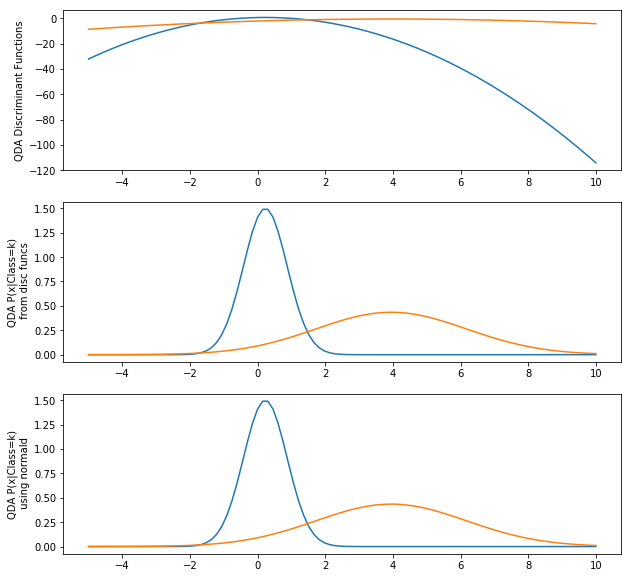Since there are only 10 training samples per class, results will change a bit from run to run.

But, what if we have more dimensions than samples? Setting $D=20$, with $N=10$, results in

In :
D = 20  # number of components in each sample
N = 10  # number of samples in each class
X1 = np.random.normal(0.0, 1.2, (N, D))
T1 = np.array(*N).reshape((N, 1))
X2 = np.random.normal(4.0, 1.8, (N, D))  # wider variance
T2 = np.array(*N).reshape((N, 1))

data = np.hstack(( np.vstack((X1, X2)), np.vstack((T1, T2))))
X = data[:, 0:D]
T = data[:, -1]
means, stds = np.mean(X,0), np.std(X,0)
Xs = (X-means)/stds

class1rows = T==1
class2rows = T==2

mu1 = np.mean(Xs[class1rows,:],axis=0)
mu2 = np.mean(Xs[class2rows,:],axis=0)

Sigma1 = np.cov(Xs[class1rows,:].T)
Sigma2 = np.cov(Xs[class2rows,:].T)

N1 = np.sum(class1rows)
N2 = np.sum(class2rows)
N = len(T)
prior1 = N1 / float(N)
prior2 = N2 / float(N)

nNew = 100
newData = np.linspace(-5.0,10.0,nNew).repeat(D).reshape((nNew,D))

d1 = discQDA(newData,means,stds,mu1,Sigma1,prior1)
d2 = discQDA(newData,means,stds,mu2,Sigma2,prior2)

plt.figure(figsize=(10,10))
plt.subplot(3,1,1)
plt.plot(newData[:,0],np.hstack((d1,d2)))
plt.ylabel("QDA Discriminant Functions")
# Plot generative distributions  p(x | Class=k)  starting with discriminant functions
plt.subplot(3,1,2)
probs = np.exp( np.hstack((d1,d2)) - 0.5*D*np.log(2*np.pi) - np.log(np.array([[prior1,prior2]])))
plt.plot(newData[:,0],probs)
plt.ylabel("QDA P(x|Class=k)\n from disc funcs", multialignment="center")

# Plot generative distributions  p(x | Class=k)  using normald
plt.subplot(3,1,3)
newDataS = (newData-means)/stds
probs = np.hstack((normald(newDataS,mu1,Sigma1),
normald(newDataS,mu2,Sigma2)))
plt.plot(newData[:,0],probs)
plt.ylabel("QDA P(x|Class=k)\n using normald", multialignment="center");

/s/parsons/e/fac/anderson/anaconda3/lib/python3.6/site-packages/ipykernel_launcher.py:11: RuntimeWarning: invalid value encountered in log
# This is added back by InteractiveShellApp.init_path()
/s/parsons/e/fac/anderson/anaconda3/lib/python3.6/site-packages/ipykernel_launcher.py:11: RuntimeWarning: invalid value encountered in sqrt
# This is added back by InteractiveShellApp.init_path()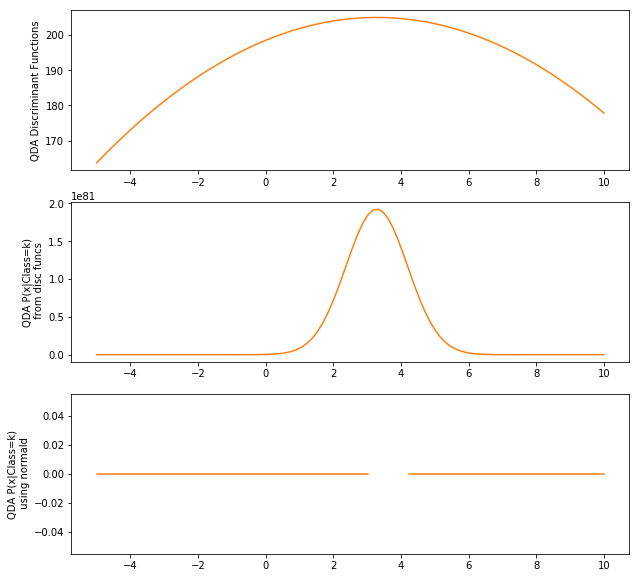What happened? $\Sigma$ is very close to singular, meaning columns of $\Xv$ are close to collinear. The determinant of a singular matrix is zero and its inverse doesn't exist. We will discuss ways of handling this in the future.

For two dimensional data from two classes, our data and decision boundary, where $\delta_1(\xv) = \delta_2(\xv)$, might look likeAssuming a single Normal distribution as the model of data from each class does not seem to lead to an exceedingly complex model. But, how many parameters are there in the mean and covariance matrix, if data is $d$-dimensional?

• Mean has $d$ components.
• Covariance matrix has $d^2$ components. If $d = 100$, the covariance matrix has 100,000 parameters. Whoa!

Actually the covariance matrix is symmetric, so it only has $\frac{d^2}{2} + \frac{d}{2} = \frac{d(d+1)}{2}$ unique values. Still a lot. And we have one for each class, so total number of parameters, including mean, is $K(d + \frac{d(d+1)}{2})$.

What if the data distribution is under-sampled?Normal distribution Gaussian for Class 1 is far from correct. Class boundary will now lead to many errors.

How can we reduce the chance of overfitting? Need to remove flexibility from the Normal distribution model. How?

Could restrict all covariance matrices to be diagonal. The ellipses would be parallel to the axes. Wouldn't work well if features are correlated.

Could force all classes to have the same covariance matrix by averaging the covariance matrices from every class.

Seems like a bad idea, but at least we are using all of the data samples to come up with a covariance matrix.

If we use the average of the covariance matrix for each class, weighted by the fraction of samples from that class, we would seeBetter result than using unique covariance matrices.

Notice the boundary. It is now linear, not the quadratic curve we had before. Why?

Remember our discriminant function.

\begin{align*} \delta_k(\xv) = -\frac{1}{2} \ln |\Sigma_k| -\frac{1}{2}(\xv-\muv_k)^T \Sigma_k^{-1} (\xv-\muv_k) + \ln P(C=k) \end{align*}

When we compare discriminant functions, $\delta_2(\xv) > \delta_1(\xv)$, and use the same covariance matrix $\Sigmav$ for every class, we get

\begin{align*} -\frac{1}{2} & \ln |\Sigma| + -\frac{1}{2}(\xv-\muv_2)^T \Sigma^{-1} (\xv-\muv_2) + \ln p(C=2) \\ & > -\frac{1}{2} \ln |\Sigma| + -\frac{1}{2}(\xv-\muv_1)^T \Sigma^{-1} (\xv-\muv_1) + \ln p(C=1) \end{align*}

which can be simplified to

\begin{align*} -\frac{1}{2}(\xv-\muv_2)^T \Sigma^{-1} (\xv-\muv_2) + \ln p(C=2) & > -\frac{1}{2}(\xv-\muv_1)^T \Sigma^{-1} (\xv-\muv_1) + \ln p(C=1) \\ \xv^T \Sigmav^{-1} \muv_1 - \frac{1}{2}\muv_1^T \Sigmav^{-1} \muv_1 + \log P(C=1) &> \xv^T \Sigmav^{-1} \muv_2 - \frac{1}{2}\muv_2^T \Sigmav^{-1} \muv_2 + \log P(C=2) \end{align*}

So, our discriminant function has become

\begin{align*} \delta_k(\xv) = \xv^T \Sigmav^{-1} \muv_k - \frac{1}{2}\muv_k^T \Sigmav^{-1} \muv_k + \log P(C=k) \end{align*}

This is linear in $\xv$, hence and can be written as

\begin{align*} \delta_k(\xv) = \xv^T \wv_k + \text{constant}_k \end{align*}

So, using Normal distributions as generative models and restricting the covariance matrices to all be the weighted average of class covariance matrices

\begin{align*} \Sigmav = \sum_{k=1}^K \frac{N_k}{N} \Sigmav_k \end{align*}

results in a linear boundary. This approach is called Linear Discriminant Analysis (LDA).

Both QDA and LDA are based on Normal distributions for modeling the data samples in each class.

QDA is more flexible, but LDA often works better in practice. When?

• Undersampled data
• Small number of samples
• High dimensional data

## Example¶

Let's play with the parkinsons data and classify it using QDA.

Calculate means and covariance matrices

In :
Xtrain.shape, Ttrain.shape, Xtest.shape, Ttest.shape

Out:
((156, 22), (156, 1), (39, 22), (39, 1))
In :
# Fit generative models (Normal distributions) to each class
means,stds = np.mean(Xtrain, 0), np.std(Xtrain, 0)
Xtrains = (Xtrain - means) / stds

Ttr = (Ttrain==0).reshape((-1))
mu1 = np.mean(Xtrains[Ttr, :], axis=0)
cov1 = np.cov(Xtrains[Ttr, :].T)
Ttr = (Ttrain.ravel()==1).reshape((-1))
mu2 = np.mean(Xtrains[Ttr, :],axis=0)
cov2 = np.cov(Xtrains[Ttr, :].T)

In :
d1 = discQDA(Xtrain, means, stds, mu1, cov1, float(nHealthy)/(nHealthy+nPark))
d2 = discQDA(Xtrain, means, stds, mu2, cov2, float(nPark)/(nHealthy+nPark))
predictedTrain = np.argmax(np.hstack((d1, d2)), axis=1)

d1t = discQDA(Xtest, means, stds, mu1, cov1, float(nHealthy)/(nHealthy+nPark))
d2t = discQDA(Xtest, means, stds, mu2, cov2, float(nPark)/(nHealthy+nPark))
predictedTest = np.argmax(np.hstack((d1t, d2t)), axis=1)

def percentCorrect(p, t):
return np.sum(p.ravel()==t.ravel()) / float(len(t)) * 100

print('Percent correct: Train', percentCorrect(predictedTrain,Ttrain), 'Test', percentCorrect(predictedTest,Ttest))

Percent correct: Train 99.35897435897436 Test 89.74358974358975


Let's write a function to do this and run it multiple times (for different divisions into training and testing sets).

In :
def runPark(filename, trainFraction):
f = open(filename,"r")

targetColumn = names.index("status")
XColumns = np.arange(23)
XColumns = np.delete(XColumns, targetColumn)
X = data[:, XColumns]
T = data[:, targetColumn].reshape((-1,1)) # to keep 2-d matrix form
names.remove("status")

healthyI,_ = np.where(T == 0)
parkI,_ = np.where(T == 1)
healthyI = np.random.permutation(healthyI)
parkI = np.random.permutation(parkI)

nHealthy = round(trainFraction*len(healthyI))
nPark = round(trainf*len(parkI))
rowsTrain = np.hstack((healthyI[:nHealthy], parkI[:nPark]))
Xtrain = X[rowsTrain, :]
Ttrain = T[rowsTrain, :]
rowsTest = np.hstack((healthyI[nHealthy:], parkI[nPark:]))
Xtest =  X[rowsTest, :]
Ttest =  T[rowsTest, :]

means, stds = np.mean(Xtrain, 0), np.std(Xtrain, 0)
Xtrains = (Xtrain-means)/stds

Ttr = (Ttrain==0).reshape((-1))
mu1 = np.mean(Xtrains[Ttr, :], axis=0)
cov1 = np.cov(Xtrains[Ttr, :].T)
Ttr = (Ttrain.ravel()==1).reshape((-1))
mu2 = np.mean(Xtrains[Ttr, :],axis=0)
cov2 = np.cov(Xtrains[Ttr, :].T)

d1 = discQDA(Xtrain, means, stds, mu1, cov1, float(nHealthy)/(nHealthy+nPark))
d2 = discQDA(Xtrain, means, stds, mu2, cov2, float(nPark)/(nHealthy+nPark))
predictedTrain = np.argmax(np.hstack((d1, d2)), axis=1)

d1t = discQDA(Xtest, means, stds, mu1, cov1, float(nHealthy)/(nHealthy+nPark))
d2t = discQDA(Xtest, means, stds, mu2, cov2, float(nPark)/(nHealthy+nPark))
predictedTest = np.argmax(np.hstack((d1t, d2t)), axis=1)

print('Percent correct: Train', percentCorrect(predictedTrain, Ttrain), 'Test', percentCorrect(predictedTest,Ttest))

def percentCorrect(p, t):
return np.sum(p.ravel()==t.ravel()) / float(len(t)) * 100

In :
runPark('parkinsons.data', 0.8)

Percent correct: Train 97.43589743589743 Test 92.3076923076923

In :
runPark('parkinsons.data',0.8)

Percent correct: Train 99.35897435897436 Test 92.3076923076923

In :
runPark('parkinsons.data',0.8)

Percent correct: Train 98.07692307692307 Test 94.87179487179486

In :
runPark('parkinsons.data',0.8)

Percent correct: Train 99.35897435897436 Test 84.61538461538461


Review. How would you get the values of

• $p(\xv|C=k)$
• $p(\xv)$
• $p(C=k|\xv)$
• predicted $C$ for a given $\xv$

Now, what would you change to do all of this for LDA?

## LDA: Linear Discriminant Analysis¶

So far we have only been applying QDA. Let's write a discLDA function and see if this classifier, which assumes all classes have the same covariance matrix, does better than QDA on our Parkinson's data.

Above we showed that if we assume the same covariance matrix, $\Sigmav$, for each class, where \begin{align*} \Sigmav = \sum_{k=1}^K \frac{N_k}{N} \Sigmav_k, \end{align*} our discriminant function becomes \begin{align*} \delta_k(\xv) = \xv^T \Sigmav^{-1} \muv_k - \frac{1}{2}\muv_k^T \Sigmav^{-1} \muv_k + \log P(C=k) \end{align*}

In :
import pdb

In :
def discLDA(X, means,stds, mu, Sigma, prior):
X = (X-means)/stds
if Sigma.size == 1:
Sigma = np.asarray(Sigma).reshape((1,1))
det = np.linalg.det(Sigma)
# if det == 0:
#    raise np.linalg.LinAlgError('discQDA(): Singular covariance matrix')
SigmaInv = np.linalg.pinv(Sigma)     # pinv in case Sigma is singular
mu = mu.reshape((-1,1)) # make mu a column vector
# pdb.set_trace()
return np.dot(np.dot(X,SigmaInv), mu) - 0.5 * np.dot(np.dot(mu.T,SigmaInv), mu) + np.log(prior)

In :
def runPark(filename, trainFraction):
f = open(filename,"r")

targetColumn = names.index("status")
XColumns = np.arange(23)
XColumns = np.delete(XColumns, targetColumn)
X = data[:, XColumns]
T = data[:, targetColumn].reshape((-1,1)) # to keep 2-d matrix form
names.remove("status")

healthyI,_ = np.where(T == 0)
parkI,_ = np.where(T == 1)
healthyI = np.random.permutation(healthyI)
parkI = np.random.permutation(parkI)

nHealthy = round(trainFraction*len(healthyI))
nPark = round(trainf*len(parkI))
rowsTrain = np.hstack((healthyI[:nHealthy], parkI[:nPark]))
Xtrain = X[rowsTrain, :]
Ttrain = T[rowsTrain, :]
rowsTest = np.hstack((healthyI[nHealthy:], parkI[nPark:]))
Xtest =  X[rowsTest, :]
Ttest =  T[rowsTest, :]

means,stds = np.mean(Xtrain,0), np.std(Xtrain,0)
Xtrains = (Xtrain-means)/stds

Ttr = (Ttrain==0).reshape((-1))
mu1 = np.mean(Xtrains[Ttr, :],axis=0)
cov1 = np.cov(Xtrains[Ttr, :].T)
Ttr = (Ttrain.ravel()==1).reshape((-1))
mu2 = np.mean(Xtrains[Ttr, :],axis=0)
cov2 = np.cov(Xtrains[Ttr, :].T)

d1 = discQDA(Xtrain, means, stds, mu1, cov1, float(nHealthy)/(nHealthy+nPark))
d2 = discQDA(Xtrain, means, stds, mu2, cov2, float(nPark)/(nHealthy+nPark))
predictedTrain = np.argmax(np.hstack((d1, d2)),axis=1)

d1t = discQDA(Xtest, means, stds, mu1, cov1, float(nHealthy)/(nHealthy+nPark))
d2t = discQDA(Xtest, means, stds, mu2, cov2, float(nPark)/(nHealthy+nPark))
predictedTest = np.argmax(np.hstack((d1t, d2t)), axis=1)

print('QDA Percent correct: Train', percentCorrect(predictedTrain, Ttrain), 'Test', percentCorrect(predictedTest,Ttest))

covMean = (cov1 * nHealthy + cov2 * nPark) / (nHealthy+nPark)
d1 = discLDA(Xtrain, means, stds, mu1, covMean, float(nHealthy)/(nHealthy+nPark))
d2 = discLDA(Xtrain, means, stds, mu2, covMean, float(nPark)/(nHealthy+nPark))
predictedTrain = np.argmax(np.hstack((d1, d2)), axis=1)

d1t = discLDA(Xtest, means, stds, mu1, covMean, float(nHealthy)/(nHealthy+nPark))
d2t = discLDA(Xtest, means, stds, mu2, covMean, float(nPark)/(nHealthy+nPark))
predictedTest = np.argmax(np.hstack((d1t, d2t)), axis=1)
print('LDA Percent correct: Train', percentCorrect(predictedTrain, Ttrain), 'Test', percentCorrect(predictedTest,Ttest))

def percentCorrect(p, t):
return np.sum(p.ravel()==t.ravel()) / float(len(t)) * 100

In :
runPark('parkinsons.data', 0.8)

QDA Percent correct: Train 96.7948717948718 Test 87.17948717948718
LDA Percent correct: Train 89.74358974358975 Test 87.17948717948718

In :
for i in range(5):
runPark('parkinsons.data', 0.8)
print()

QDA Percent correct: Train 98.07692307692307 Test 87.17948717948718
LDA Percent correct: Train 91.66666666666666 Test 89.74358974358975

QDA Percent correct: Train 97.43589743589743 Test 92.3076923076923
LDA Percent correct: Train 91.66666666666666 Test 87.17948717948718

QDA Percent correct: Train 98.07692307692307 Test 89.74358974358975
LDA Percent correct: Train 89.74358974358975 Test 84.61538461538461

QDA Percent correct: Train 99.35897435897436 Test 82.05128205128204
LDA Percent correct: Train 91.02564102564102 Test 89.74358974358975

QDA Percent correct: Train 98.07692307692307 Test 82.05128205128204
LDA Percent correct: Train 92.94871794871796 Test 84.61538461538461


In :
import sys
sys.float_info.epsilon, np.log(sys.float_info.epsilon)

Out:
(2.220446049250313e-16, -36.04365338911715)
In :
%%writefile qdalda.py

import numpy as np
import sys # for sys.float_info.epsilon

######################################################################
### class QDA
######################################################################

class QDA(object):

def __init__(self):
# Define all instance variables here. Not necessary
self.means = None
self.stds = None
self.mu = None
self.sigma = None
self.sigmaInv = None
self.prior = None
self.determinant = None
self.discriminantConstant = None

def train(self, X, T):
self.classes = np.unique(T)
self.means, self.stds = np.mean(X,0), np.std(X,0)
Xs = (X - self.means) / self.stds
self.mu = []
self.sigma = []
self.sigmaInv = []
self.determinant = []
self.prior = []
nSamples = X.shape
for k in self.classes:
rowsThisClass = (T == k).reshape((-1))
self.mu.append( np.mean(Xs[rowsThisClass, :], 0).reshape((-1,1)) )
self.sigma.append( np.cov(Xs[rowsThisClass, :], rowvar=0) )
if self.sigma[-1].size == 1:
self.sigma[-1] = self.sigma[-1].reshape((1,1))
det = np.linalg.det(self.sigma[-1])
if det == 0:
det = sys.float_info.epsilon
self.determinant.append( det )
self.sigmaInv.append( np.linalg.pinv(self.sigma[-1]) )    # pinv in case Sigma is singular
self.prior.append( np.sum(rowsThisClass) / float(nSamples) )
self._finishTrain()

def _finishTrain(self):
self.discriminantConstant = []
for ki in range(len(self.classes)):
self.discriminantConstant.append( np.log(self.prior[ki]) - 0.5*np.log(self.determinant[ki]) )

def use(self, X, allOutputs=False):
nSamples = X.shape
Xs = (X - self.means) / self.stds
discriminants,probabilities = self._discriminantFunction(Xs)
predictedClass = self.classes[np.argmax( discriminants, axis=1 )]
predictedClass = predictedClass.reshape((-1, 1))
return (predictedClass, probabilities, discriminants) if allOutputs else predictedClass

def _discriminantFunction(self, Xs):
nSamples = Xs.shape
discriminants = np.zeros((nSamples, len(self.classes)))
for ki in range(len(self.classes)):
Xc = Xs - self.mu[ki].T
discriminants[:,ki:ki+1] = self.discriminantConstant[ki] - 0.5 * \
np.sum(np.dot(Xc, self.sigmaInv[ki]) * Xc, axis=1).reshape((-1,1))
D = Xs.shape
probabilities = np.exp( discriminants - 0.5*D*np.log(2*np.pi) )
return discriminants, probabilities

def __repr__(self):
if self.mu is None:
return 'QDA not trained.'
else:
return 'QDA trained for classes {}'.format(self.classes)

######################################################################
### class LDA
######################################################################

class LDA(QDA):

def _finishTrain(self):
self.sigmaMean = np.sum(np.stack(self.sigma) * np.array(self.prior)[:,np.newaxis,np.newaxis], axis=0)
self.sigmaMeanInv = np.linalg.pinv(self.sigmaMean)
# print(self.sigma)
# print(self.sigmaMean)
self.discriminantConstant = []
self.discriminantCoefficient = []
for ki in range(len(self.classes)):
sigmaMu = np.dot(self.sigmaMeanInv, self.mu[ki])
self.discriminantConstant.append( -0.5 * np.dot(self.mu[ki].T, sigmaMu) )
self.discriminantCoefficient.append( sigmaMu )

def _discriminantFunction(self,Xs):
nSamples = Xs.shape
discriminants = np.zeros((nSamples, len(self.classes)))
for ki in range(len(self.classes)):
discriminants[:,ki:ki+1] = self.discriminantConstant[ki] + \
np.dot(Xs, self.discriminantCoefficient[ki])
D = Xs.shape
probabilities = np.exp( discriminants - 0.5*D*np.log(2*np.pi) - 0.5*np.log(self.determinant[ki]) \
- 0.5*np.sum(np.dot(Xs,self.sigmaMeanInv) * Xs, axis=1).reshape((-1,1)))
return discriminants, probabilities

######################################################################
### Example use
######################################################################

if __name__ == '__main__':

D = 1  # number of components in each sample
N = 10  # number of samples in each class
X = np.vstack((np.random.normal(0.0, 1.0, (N, D)),
np.random.normal(4.0, 1.5, (N, D))))
T = np.vstack((np.array(*N).reshape((N, 1)),
np.array(*N).reshape((N, 1))))

qda = QDA()
qda.train(X,T)
c,prob,_ = qda.use(X, allOutputs=True)
print('QDA', np.sum(c==T)/X.shape * 100, '% correct')
print('{:>3s} {:>4s} {:>14s}'.format('T','Pred','prob(C=k|x)'))
for row in np.hstack((T,c,prob)):
print('{:3.0f} {:3.0f} {:8.4f} {:8.4f}'.format(*row))

lda = LDA()
lda.train(X,T)
c,prob,d = lda.use(X, allOutputs=True)
print('LDA', np.sum(c==T)/X.shape * 100, '% correct')
print('{:>3s} {:>4s} {:>14s}'.format('T','Pred','prob(C=k|x)'))
for row in np.hstack((T,c,prob)):
print('{:3.0f} {:3.0f} {:8.4f} {:8.4f}'.format(*row))

Overwriting qdalda.py

In :
%run qdalda.py

QDA 90.0 % correct
T Pred    prob(C=k|x)
1   1   0.4174   0.0182
1   1   0.1992   0.0645
1   1   0.3500   0.0300
1   1   0.2238   0.0574
1   1   0.0530   0.0001
1   1   0.4415   0.0133
1   1   0.4315   0.0052
1   1   0.4327   0.0053
1   1   0.3110   0.0016
1   1   0.4363   0.0057
2   2   0.0000   0.1612
2   1   0.1131   0.0997
2   2   0.0012   0.2978
2   2   0.0010   0.3008
2   2   0.0001   0.3025
2   2   0.0000   0.2886
2   1   0.1306   0.0907
2   2   0.0000   0.2841
2   2   0.0005   0.3084
2   2   0.0000   0.0925
LDA 90.0 % correct
T Pred    prob(C=k|x)
1   1   0.5908   0.0132
1   1   0.3672   0.0737
1   1   0.5275   0.0260
1   1   0.3957   0.0628
1   1   0.1566   0.0000
1   1   0.6125   0.0086
1   1   0.6035   0.0024
1   1   0.6047   0.0025
1   1   0.4889   0.0005
1   1   0.6079   0.0027
2   2   0.0000   0.2552
2   1   0.2551   0.1329
2   2   0.0140   0.5868
2   2   0.0124   0.5949
2   2   0.0022   0.5995
2   2   0.0012   0.5624
2   1   0.2799   0.1169
2   2   0.0010   0.5504
2   2   0.0079   0.6152
2   2   0.0000   0.1201

In [ ]: